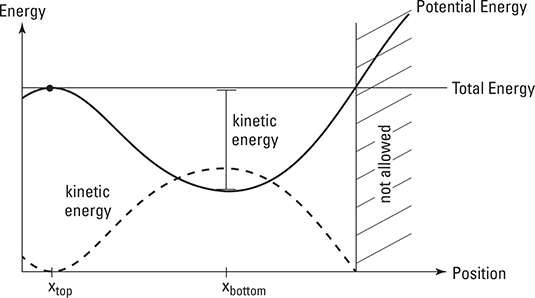##### String Theory For DummiesIn physics, the potential energy of an object depends on its position. A boulder has more potential energy when it’s at the top of a hill than when it’s rolling down. In your physics class, you may be asked to interpret or draw an energy diagram. An energy diagram shows how the potential energy of an object depends on position and tells you all kinds of things about the motion of the object.

Here’s an example energy diagram for the boulder:The potential energy curve shows how much potential energy the boulder has at each position. The boulder has more gravitational potential energy higher up the hill, so the curve also shows the shape of the hill.

But what is that horizontal line going straight across the diagram? That’s the total energy of the boulder. If the boulder is sitting precariously at the top of the hill at xtop, its potential energy is equal to its total energy. A slight breeze gives the boulder a nudge, and it starts rolling down the hill. When it reaches the bottom of the hill at xbottom, it has less potential energy. The rest of its energy is kinetic energy, and you can read exactly how much kinetic energy the boulder has from the diagram — the kinetic energy is just the distance between the potential energy curve and total energy line.

As you watch the boulder roll up the other hill toward you, you wonder how high the boulder will roll. You know the boulder will stop when its kinetic energy is zero, or when the total energy is equal to the potential energy. You go to the place where the potential energy curve and total energy line cross, and take one more step up the hill. As you turn around, you see the boulder slow down, momentarily stop just in front of you, and roll away back down the hill. Phew!

Here’s what you should keep in mind about energy diagrams:

• The total energy doesn’t change. The height of the potential energy curve is the potential energy of the object, and the distance between the potential energy curve and the total energy line is the kinetic energy of the object.

• The object will turn around where the total energy line and potential energy curve cross.

• If you start the object at a different location or with a different initial kinetic energy, the total energy line can shift up or down. The potential energy curve is a property of the object and whatever it’s interacting with.

• The object can never be at a location where the potential energy curve is above the total energy line.

• The object feels a force pulling it down the slope toward the location with lower potential energy.